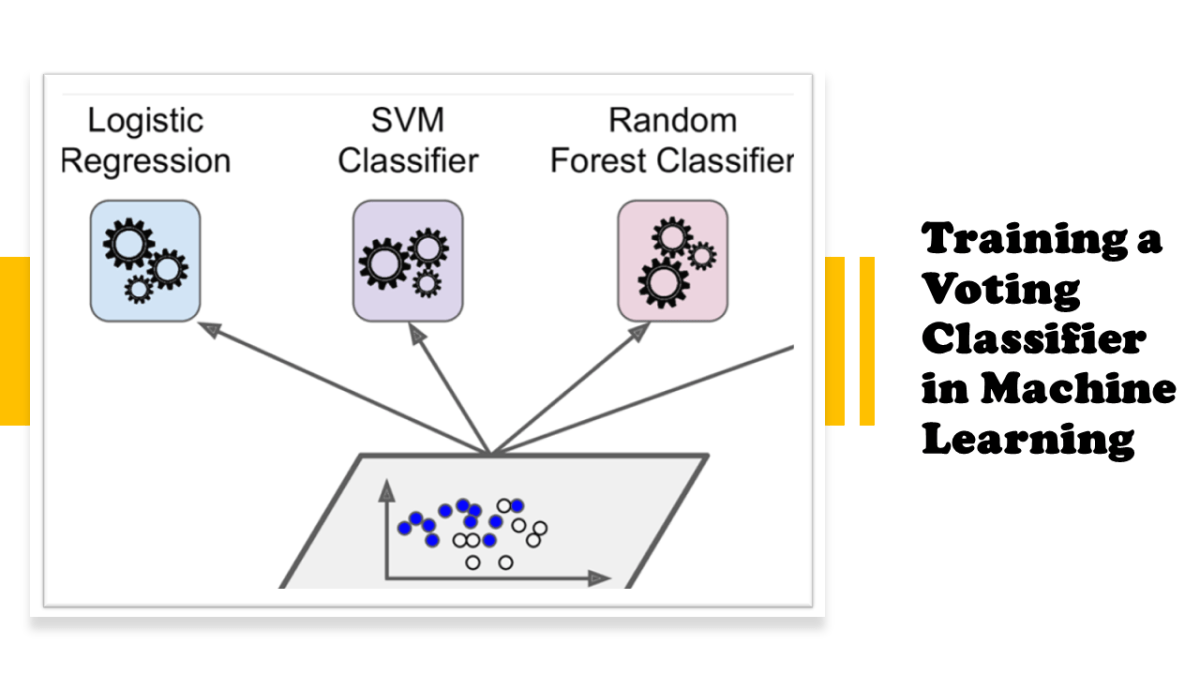# Voting Classifier in Machine Learning`import sysassert sys.version_info >= (3, 5)# Scikit-Learn ≥0.20 is requiredimport sklearnassert sklearn.__version__ >= "0.20"# Common importsimport numpy as npimport os# to make this notebook's output stable across runsnp.random.seed(42)# To plot pretty figures%matplotlib inlineimport matplotlib as mplimport matplotlib.pyplot as pltmpl.rc('axes', labelsize=14)mpl.rc('xtick', labelsize=12)mpl.rc('ytick', labelsize=12)`